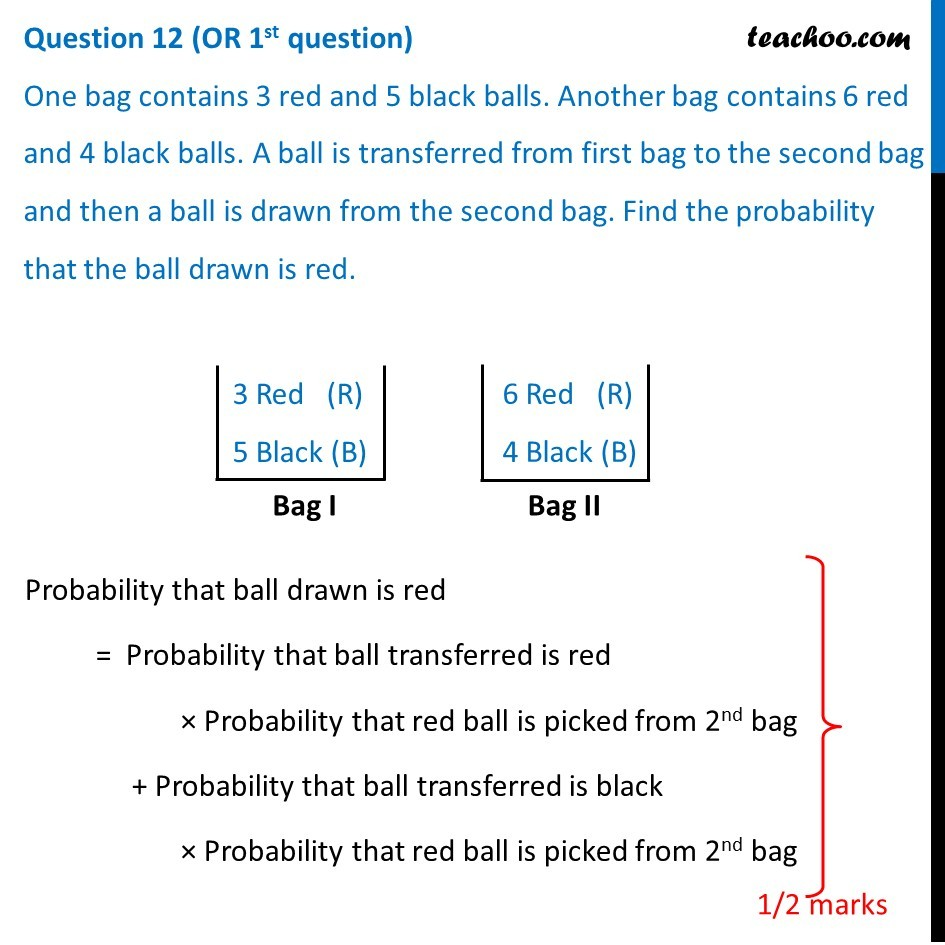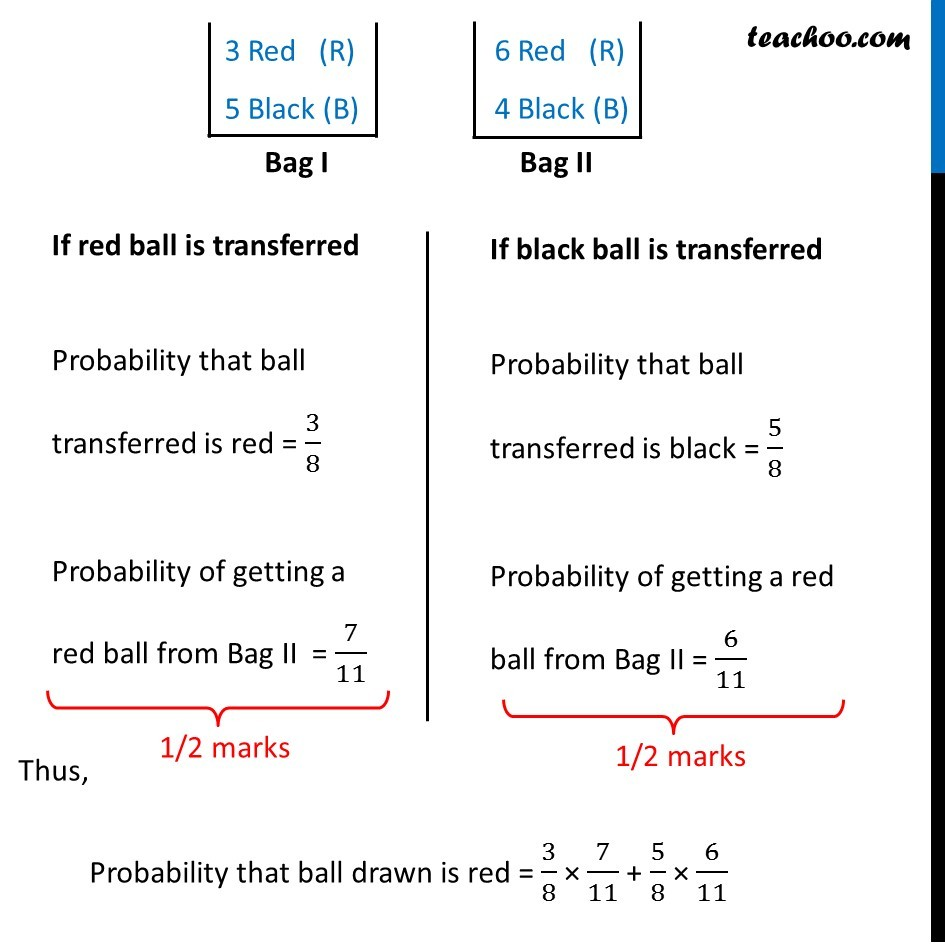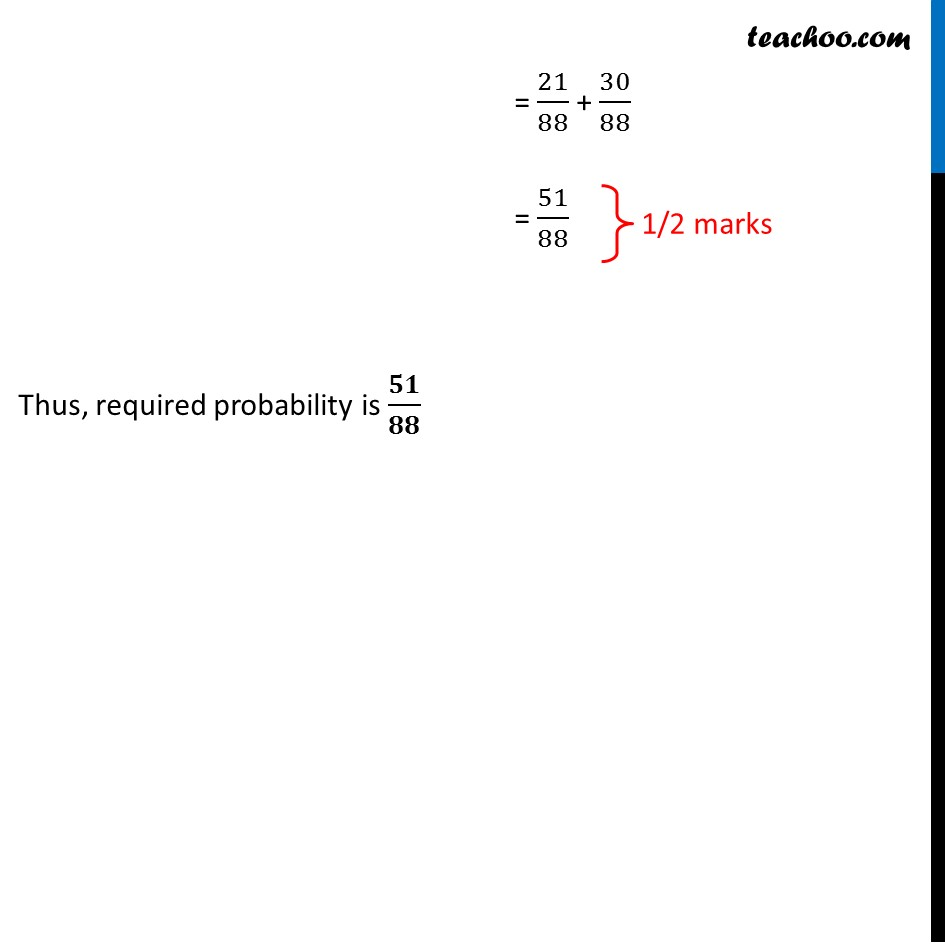CBSE Class 12 Sample Paper for 2019 Boards

Class 12
Solutions of Sample Papers and Past Year Papers - for Class 12 Boards

## One bag contains 3 red and 5 black balls. Another bag contains 6 red and 4 black balls. A ball is transferred from first bag to the second bag and then a ball is drawn from the second bag. Find the probability that the ball drawn is red.Learn in your speed, with individual attention - Teachoo Maths 1-on-1 Class

### Transcript

Question 12 (OR 1st question) One bag contains 3 red and 5 black balls. Another bag contains 6 red and 4 black balls. A ball is transferred from first bag to the second bag and then a ball is drawn from the second bag. Find the probability that the ball drawn is red. 3 Red (R) 5 Black (B) 6 Red (R) 4 Black (B) Probability that ball drawn is red = Probability that ball transferred is red × Probability that red ball is picked from 2nd bag + Probability that ball transferred is black × Probability that red ball is picked from 2nd bag 3 Red (R) 5 Black (B) 6 Red (R) 4 Black (B) If red ball is transferred Probability that ball transferred is red = 3/8 Probability of getting a red ball from Bag II = 7/11 If black ball is transferred Probability that ball transferred is black = 5/8 Probability of getting a red ball from Bag II = 6/11 Thus, Probability that ball drawn is red = 3/8 × 7/11 + 5/8 × 6/11 = 21/88 + 30/88 = 51/88 Thus, required probability is 𝟓𝟏/𝟖𝟖